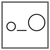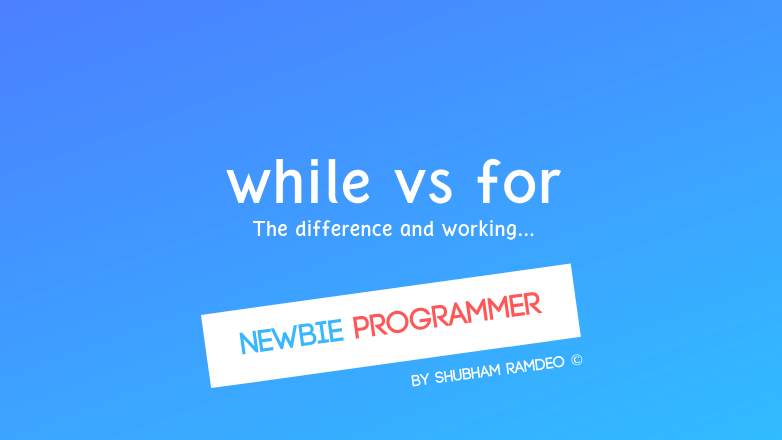Fuddlepixel coz tech is fun();

# Different kinds of loop

Let us discuss and differentiate between the different kinds of loop, namely for, while and do while available in C language.

By Shubham Ramdeo
28 July 2015 · 10 mins read
# `c`  `newbie`Let’s discuss different types of loop available in C language !

Welcome back to the Newbie Programmer Series. In the last part (click here) we have learnt about the theory of looping. What is loop and how to use loops in C language. Now we know how to use for and while and do - while loops in C, which I have called them as Direct Methods of loops. But still, even if we know the concept, we have not used them practically. But there is a question that I want to deal with in this part. And that is, what is the difference between While, Do While and For Loop ? If they work same, why do they all exist ? That means there is some difference between them. And we are gonna learn about that in this part. So if you are new to this series, please go to the index (click here) and read out all the previous parts so that you can easily understand what is happening below.

## Theoretically

We have already discussed in the previous part that these loops have same parameters ( check out the previous post here ), works same but still they exist differently. If both of them are same then why they exist separately ? Well for a Newbie Programmer like us, at first we would find no difference. But an experienced programmer will find a little but important difference in their application.

There is a technical difference between their definitions. The one that we have learnt in the previous part was for quick understanding.

### While, to search for the truth

The real definition of while loop is like:

while ( true ) { instructions to repeat }

That is, the while loop will repeat itself until the condition written inside while is true. In computer science, true means 1. So the loop will run until it finds the condition is satisfied. This condition needs not to be a mathematical one. Here there is no need of a counter variable as needed for the for loop. I mean, loop in not necessary to be counted. Just until a certain condition is not satisfied, it will be looped !

### For, the counter

for (counter is not stop) { instructions to repeat }

That is, the for loop is actually based on a counter and not on a condition that needs to be true. When the loop starts, the counter starts, and when the counter limit is reached, loop stops and the program comes out of the brackets. Here the counter variable changes its value each time the loop is repeated.

I had a great difficulty understanding this difference but then I created these examples below which will tell you everything:

The real application of while is like:

``````while ( john is my good friend )
{
help john
}
``````

and the real application of for is like:

``````for ( 30 days )
{
take diet food only
}
``````

### Do While ? Learn after repeating !

Yes. Do-While can be said as a loop that learns after repeating. What does it mean ? How while loop works is that it will search for the condition. If its true, then it will repeat it. In the case with do-while, It will repeat the block once first, then it will check for the condition. as the syntax says

do { this; } while (condition is true)

So it will first repeat, and then will check. Even if there is any mistake in the repeating part, which is not true with the condition, still the loop will be performed once. But not second time. Loop will be executed at least once. You will learn with the examples below. That’s the little but the great difference that I wanted to tell you. Because such little facts are later going to help us a lot.

Lets check this practically

## Practically

### While loop, satisfying true conditions

So we have learnt that while loop while continue if the condition is TRUE. Hence let’s do some example to test that.

``````#include <stdio.h>
main()
{
int x = 9999;
while ( x > 9 )
{
printf(" 1 digit number to stop, rest to repeat \n");
scanf("%d",&x);
}
printf("Bye \n");
}
``````

Lets check out line by line how this program works. First of all we tell the program to use stdio.h file which contain the printf and scanf commands. Then our main program starts. A variable x is declared with a value of 9999. Actually whenever we declare a variable it takes any random value. But I want to have x any value greater than 9 so I simply pre assigned it 9999. You can give it any other value but greater that 9. Now, the program comes to the while loop. The condition written there to continue the loop is “x > 9” and we have x = 9999 which is already greater than 9 so the loop will continue. Now, it will ask you “ 1 digit number to stop, rest to repeat “ That means that now you are gonna enter a number. If you entered a single digit number or if it is less than 10, the program will stop. And if your number is greater than 9 or any two, three, four any greater digit number ( and not a single digit ) the program will continue to ask you the same question. Now there is scanf command which make you to enter an integer value ( we have used %d ) into the variable x. Suppose you have entered the number 21 and pressed enter. You can see that after the scanf command, the loop body ends so now its time to check whether loop should repeat or not. Program again finds the condition that x > 9 but you have entered 21 which is greater than 9 so the condition is satisfied and hence the loop body will repeat again. Lets this time if you entered 5 and pressed enter, the program would find that the condition x > 9 is no longer true now as you have entered 5 which is less than 9 so the loop body will end. Out of the loop body now, there exist a ‘Bye” message. The program will say you bye and will now end.

That was the real working behind a while loop.

### For loop, waiting till the counter stops.

Writing hello world 10 times is the easiest self explanatory example.

``````#include <stdio.h>
main()
{
int x;
for ( x = 0; x < 10; x++ )
{
printf("Hello world \n ");
}
}
``````

for loop has no emotions about the conditions. It just want to reach its counter limit. Here above we have given x to go from 0 to 9. As x is an integer, its starting from 0 and ends before reaching 10 i.e at 9 so its counting from 0 to 9. At first x is 0, it will write hello world and x becomes 2 and again it writes hello world. This process continues till x becomes 9. After 9, x will go to 10 but that is out of the limit of our counter so the loop ends.

Lets do an another example

``````#include<stdio.h>
main()
{
int x;
printf("Squares \n");
for (x =1; x < 11; x++ )
{
printf("Square of %d is %d \n", x, x*x );
}
printf ("table ends");
}
``````

OUTPUT:

``````Squares
Square of 1 is 1
Square of 2 is 4
Square of 3 is 9
Square of 4 is 16
Square of 5 is 25
Square of 6 is 36
Square of 7 is 49
Square of 8 is 64
Square of 9 is 81
Square of 10 is 100
table ends
``````

lets check out its working. You already know until variable x is declared. Now a message will be printed “Squares” just as name of the program. Then the loop starts. Do you find a difference from while ? In for loop, we don’t need to assign a value to the counting variable separately as for loop already has a parameter for that. Here we have assigned x = 1. The loop will continue from 1 to 10 that is 10 times. The loop starts from x = 1. Then it prints “Square of %d is %d” and for these %d, we have the values of x and xx respectively. That means “Square of x is xx” For now when x = 1,  it becomes, “Square of 1 is 1” and the loop ends but still the loop has to repeat 9 more times. The loop has yet repeated 1 time so the counter variable, x was 1, now its second repetition so now the x is 2. Th program again repeats but with x = 2 so it prints “Square of 2 is 4”. This process continues until x becomes 10. After that, the counter stops and the program comes out of loop  body. There again a message “table ends” And after that the program stops.

That was the real working behind the for loop.

### Do while, learning from mistakes !

Here I will make a wrong program and will show that do-while loop repeat at least one time. I will apply a wrong condition. The program will not work if you used while only but with do while, the wrong program will run once, then check for the condition. It will found that the condition is wrong so it will stop and will not repeat second time. But in this process, at least one repetition will occur.

``````#include <stdio.h>
main()
{
int x = 10;
do
{
printf("Hello world \n ");
}while (x < 2);
}
``````

What is happening here is that I have assigned x to 10. And the condition for repetition is that x must be less than 2. But here x is already greater than 10 and so the condition is not met. Therefore the while loop will not work. But in the do-while loop, the program will be performed line by line, even if the condition of x < 2 is not met, still it will print hello world at least once. Then after it will check the condition, which is not true hence will not repeat further. I think that’s enough for now. We have to learn a lot of concept. I want to keep everything as simple as possible. So I have to stop now other wise our brain will go haywire for no matter. Well obviously this was a hard concept but learning such cool and little things is necessary.

So in the next part, we will discuss more applications of loops. The real fun of loop is still waiting. So stay connected.

Please share this post as much as you can so that we can get connected to more geeks who wants to learn programming but have no clue. Thanks for reading :)

##### Update me weekly ✉

Subscribe below and we’ll send you a weekly email summary of all new Code tutorials. Never miss out on learning about the next big thing.

#### Shubham RamdeoShubham Ramdeo is the founder of Fuddlepixel. He is a geek, a writer, involved with many technical and humanity projects.

Meet me!

#### Recent#### Related

`c`

`newbie`

Top